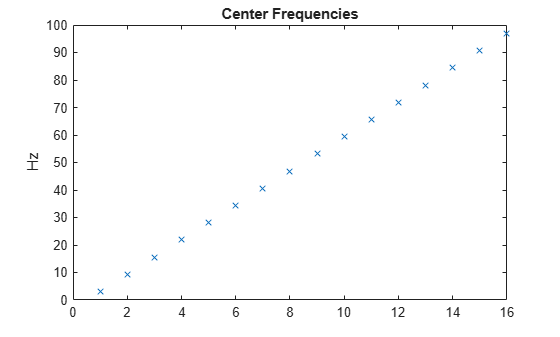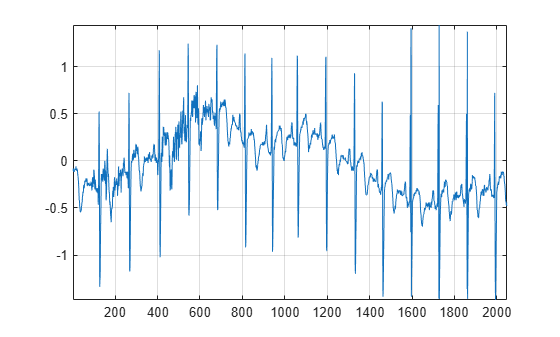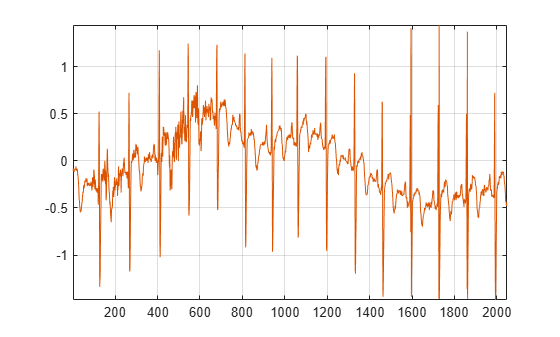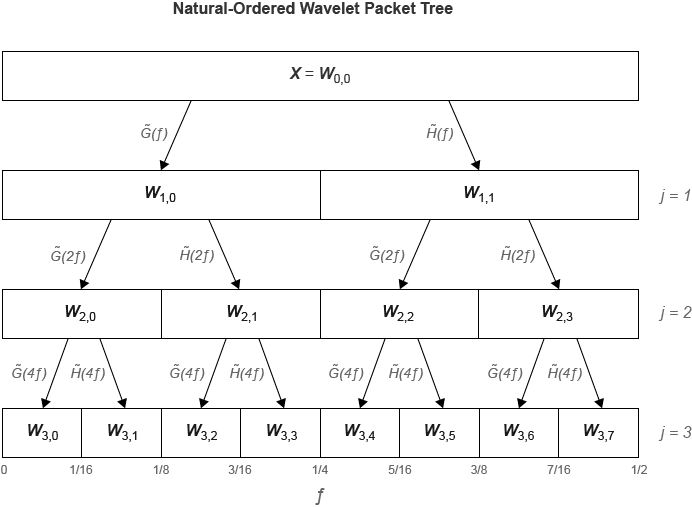# dwpt

Multisignal 1-D wavelet packet transform

## Syntax

``wpt = dwpt(X)``
``wpt = dwpt(X,wname)``
``wpt = dwpt(X,LoD,HiD)``
``[wpt,l] = dwpt(___)``
``[wpt,l,packetlevels] = dwpt(___)``
``[wpt,l,packetlevels,f] = dwpt(___)``
``[wpt,l,packetlevels,f,re] = dwpt(___)``
``[___] = dwpt(___,Name,Value)``

## Description

````wpt = dwpt(X)` returns the terminal (final-level) nodes of the discrete wavelet packet transform (DWPT) of `X`. The input `X` is a real-valued vector, matrix, or timetable. By default, the `fk18` wavelet is used, and the decomposition level is `floor(log2(Ns))`, where Ns is the number of data samples. The wavelet packet transform `wpt` is a 1-by-N cell array, where ```N = 2^floor(log2(Ns))```.```
````wpt = dwpt(X,wname)` uses the wavelet specified by `wname` for the DWPT. `wname` must be recognized by `wavemngr`.```
````wpt = dwpt(X,LoD,HiD)` uses the scaling (lowpass) filter, `LoD`, and wavelet (highpass) filter, `HiD`.```
````[wpt,l] = dwpt(___)` also returns the bookkeeping vector using any of the previous syntaxes. The vector `l` contains the length of the input signal and the number of coefficients by level. The bookkeeping vector is required for perfect reconstruction.```
````[wpt,l,packetlevels] = dwpt(___)` also returns the transform levels of the nodes of `wpt` using any of the previous syntaxes.```
````[wpt,l,packetlevels,f] = dwpt(___)` also returns the center frequencies of the approximate passbands in cycles per sample using any of the previous syntaxes.```
````[wpt,l,packetlevels,f,re] = dwpt(___)` also returns the relative energy for the wavelet packets in `wpt` using any of the previous syntaxes. The relative energy is the proportion of energy contained in each wavelet packet by level.```

example

````[___] = dwpt(___,Name,Value)` specifies options using name-value pair arguments in addition to the input arguments in the previous syntaxes. For example, `'Level',4` specifies the decomposition level.```

## Examples

collapse all

Load the 23-channel EEG data `Espiga3` . The data is sampled at 200 Hz.

`load Espiga3`

Compute the 1-D DWPT of the data using the `sym3` wavelet down to level 4. Obtain the terminal wavelet packet nodes, bookkeeping vector, and center frequencies of the approximate passbands.

`[wpt,bk,~,f] = dwpt(Espiga3,'sym3','Level',4);`

The output `wpt` is a 1-by-${2}^{4}$ cell array. Every element of `wpt` is a matrix. Choose any terminal node, and confirm the size of the matrix is 23-by-M, where M is the last element of the bookkeeping vector `bk`.

```nd = 13; size(wpt{nd})```
```ans = 1×2 23 66 ```
`bk(end)`
```ans = 66 ```

Extract the final-level coefficients of the fifth channel.

```p5 = cell2mat(cellfun(@(x) x(5,:).',wpt,'UniformOutput',false)); size(p5)```
```ans = 1×2 66 16 ```

The terminal nodes are sequency-ordered. Plot the center frequencies of the approximate passbands in hertz, and confirm they are in order of increasing frequency.

```plot(200*f,'x') title('Center Frequencies') ylabel('Hz')```This example shows how to take an expression of a biorthogonal filter pair and construct lowpass and highpass filters to produce a perfect reconstruction (PR) pair in Wavelet Toolbox™.

The LeGall 5/3 filter is the wavelet used in JPEG2000 for lossless image compression. The lowpass (scaling) filters for the LeGall 5/3 wavelet have five and three nonzero coefficients respectively. The expressions for these two filters are:

`${H}_{0}\left(z\right)=1/8\left(-{z}^{2}+2z+6+2{z}^{-1}-{z}^{-2}\right)$`

`${H}_{1}\left(z\right)=1/2\left(z+2+{z}^{-1}\right)$`

Create these filters.

```H0 = 1/8*[-1 2 6 2 -1]; H1 = 1/2*[1 2 1];```

Many of the discrete wavelet and wavelet packet transforms in Wavelet Toolbox rely on the filters being both even-length and equal in length in order to produce the perfect reconstruction filter bank associated with these transforms. These transforms also require a specific normalization of the coefficients in the filters for the algorithms to produce a PR filter bank. Use the `biorfilt` function on the lowpass prototype functions to produce the PR wavelet filter bank.

`[LoD,HiD,LoR,HiR] = biorfilt(H0,H1);`

The sum of the lowpass analysis and synthesis filters is now equal to $\sqrt{2}$.

`sum(LoD)`
```ans = 1.4142 ```
`sum(LoR)`
```ans = 1.4142 ```

The wavelet filters sum, as required, to zero. The L2-norms of the lowpass analysis and highpass synthesis filters are equal. The same holds for the lowpass synthesis and highpass analysis filters.

Now you can use these filters in discrete wavelet and wavelet packet transforms and achieve a PR wavelet packet filter bank. To demonstrate this, load and plot an ECG signal.

```load wecg plot(wecg) axis tight grid on```Obtain the discrete wavelet packet transform of the ECG signal using the LeGall 5/3 filter set.

`[wpt,L] = dwpt(wecg,LoD,HiD);`

Now use the reconstruction (synthesis) filters to reconstruct the signal and demonstrate perfect reconstruction.

```xrec = idwpt(wpt,L,LoR,HiR); plot([wecg xrec]) axis tight, grid on;````norm(wecg-xrec,'Inf')`
```ans = 3.3307e-15 ```

You can also use this filter bank in the 1-D and 2-D discrete wavelet transforms. Read and plot an image.

```im = imread('woodsculp256.jpg'); image(im); axis off;```Obtain the 2-D wavelet transform using the LeGall 5/3 analysis filters.

`[C,S] = wavedec2(im,3,LoD,HiD);`

Reconstruct the image using the synthesis filters.

```imrec = waverec2(C,S,LoR,HiR); image(uint8(imrec)); axis off;```The LeGall 5/3 filter is equivalent to the built-in `'bior2.2'` wavelet in Wavelet Toolbox. Use the `'bior2.2'` filters and compare with the LeGall 5/3 filters.

```[LD,HD,LR,HR] = wfilters('bior2.2'); subplot(2,2,1) hl = stem([LD' LoD']); hl(1).MarkerFaceColor = [0 0 1]; hl(1).Marker = 'o'; hl(2).MarkerFaceColor = [1 0 0]; hl(2).Marker = '^'; grid on title('Lowpass Analysis') subplot(2,2,2) hl = stem([HD' HiD']); hl(1).MarkerFaceColor = [0 0 1]; hl(1).Marker = 'o'; hl(2).MarkerFaceColor = [1 0 0]; hl(2).Marker = '^'; grid on title('Highpass Analysis') subplot(2,2,3) hl = stem([LR' LoR']); hl(1).MarkerFaceColor = [0 0 1]; hl(1).Marker = 'o'; hl(2).MarkerFaceColor = [1 0 0]; hl(2).Marker = '^'; grid on title('Lowpass Synthesis') subplot(2,2,4) hl = stem([HR' HiR']); hl(1).MarkerFaceColor = [0 0 1]; hl(1).Marker = 'o'; hl(2).MarkerFaceColor = [1 0 0]; hl(2).Marker = '^'; grid on title('Highpass Synthesis')```## Input Arguments

collapse all

Input data, specified as a real-valued vector, matrix, or timetable. If `X` is a matrix, the transform is applied to each column of `X`. If `X` is a timetable, `X` must either contain a matrix in a single variable or column vectors in separate variables. `X` must be uniformly sampled.

Data Types: `single` | `double`

Wavelet to use in the DWPT, specified as a character vector or string scalar. `wname` must be recognized by `wavemngr`.

You cannot specify both `wname` and a filter pair, `LoD` and `HiD`.

Example: `wpt = dwpt(data,"sym4")` specifies the `sym4` wavelet.

Wavelet analysis (decomposition) filters to use in the DWPT, specified as a pair of real-valued vectors. `LoD` is the scaling (lowpass) analysis filter, and `HiD` is the wavelet (highpass) analysis filter. You cannot specify both `wname` and a filter pair, `LoD` and `HiD`. See `wfilters` for additional information.

Note

`dwpt` does not check that `LoD` and `HiD` satisfy the requirements for a perfect reconstruction wavelet packet filter bank. See PR Biorthogonal Filters for an example of how to take a published biorthogonal filter and ensure that the analysis and synthesis filters produce a perfect reconstruction wavelet packet filter bank using `dwpt`.

### Name-Value Arguments

Specify optional pairs of arguments as `Name1=Value1,...,NameN=ValueN`, where `Name` is the argument name and `Value` is the corresponding value. Name-value arguments must appear after other arguments, but the order of the pairs does not matter.

Before R2021a, use commas to separate each name and value, and enclose `Name` in quotes.

Example: `wpt = dwpt(x,'sym4','Level',4)` specifies a level 4 decomposition using the `sym4` wavelet.

Wavelet decomposition level, specified as a positive integer less than or equal to `floor(log2(Ns))`, where Ns is the number of samples in the data. If unspecified, `Level` defaults to `floor(log2(Ns))`.

Wavelet packet tree handling, specified as a numeric or logical `1` (`true`) or `0` (`false`). When set to `true`, `wpt` contains the full packet tree. When set to `false`, `wpt` contains only the terminal nodes. If unspecified, `FullTree` defaults to `false`.

Wavelet packet transform boundary handling, specified as `'reflection'` or `'periodic'`. Setting to `'reflection'` or `'periodic'`, the wavelet packet coefficients are extended at each level based on the `'sym'` or `'per'` mode in `dwtmode`, respectively. If unspecified, `Boundary` defaults to `'reflection'`.

## Output Arguments

collapse all

Wavelet packet transform, returned as a 1-by-M cell array. If taking the DWPT of one signal, each element of `wpt` is a vector. Otherwise, each element is a matrix. The coefficients in the jth row of the matrix correspond to the signal in the jth column of `X`. The packets are sequency-ordered.

If returning the terminal nodes of a level N decomposition, `wpt` is a 1-by-2N cell array. If returning the full wavelet packet tree, `wpt` is a 1-by-(2N+1−2) cell array.

Bookkeeping vector, returned as a vector of positive integers. The vector `l` contains the length of the input signal and the number of coefficients by level, and is required for perfect reconstruction.

Transform levels, returned as a vector of positive integers. The ith element of `packetlevels` corresponds to the ith element of `wpt`. If `wpt` contains only the terminal nodes, `packetlevels` is a vector with each element equal to the terminal level. If `wpt` contains the full wavelet packet tree, then `packetlevels` is a vector with 2j elements for each level j.

Center frequencies of the approximate passbands in cycles per sample, returned as a real-valued vector. The jthe element of `f` corresponds to the jth wavelet packet node of `wpt`. You can multiply the elements in `f` by a sampling frequency to convert to cycles per unit time.

Relative energy for the wavelet packets in `wpt`, returned as a cell array. The relative energy is the proportion of energy contained in each wavelet packet by level. The jth element of `re` corresponds to the jth wavelet packet node of `wpt`.

Each element of `re` is a scalar when taking the DWPT of one signal. Otherwise, when taking the DWPT of M signals, each element of `re` is a M-by-1 vector, where the ith element is the relative energy of the ith signal channel. For each channel, the sum of relative energies in the wavelet packets at a given level is equal to 1.

## Algorithms

The `dwpt` function performs a discrete wavelet packet transform and produces a sequency-ordered wavelet packet tree. Compare the sequency-ordered and normal (Paley)-ordered trees. $\stackrel{˜}{G}\left(f\right)$ is the scaling (lowpass) analysis filter, and $\stackrel{˜}{H}\left(f\right)$ represents the wavelet (highpass) analysis filter. The labels at the bottom show the partition of the frequency axis [0, ½].Wickerhauser, Mladen Victor. Adapted Wavelet Analysis from Theory to Software. Wellesley, MA: A.K. Peters, 1994.

 Percival, D. B., and A. T. Walden. Wavelet Methods for Time Series Analysis. Cambridge, UK: Cambridge University Press, 2000.

 Mesa, Hector. “Adapted Wavelets for Pattern Detection.” In Progress in Pattern Recognition, Image Analysis and Applications, edited by Alberto Sanfeliu and Manuel Lazo Cortés, 3773:933–44. Berlin, Heidelberg: Springer Berlin Heidelberg, 2005. https://doi.org/10.1007/11578079_96.